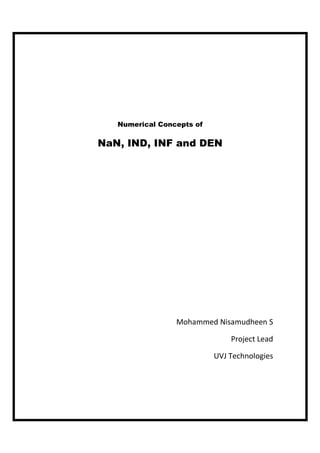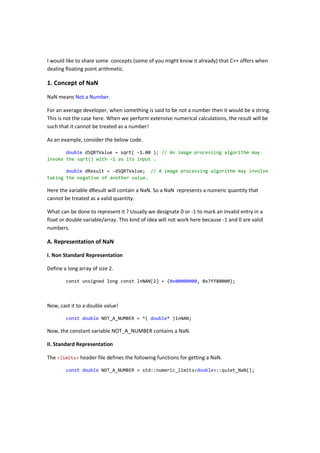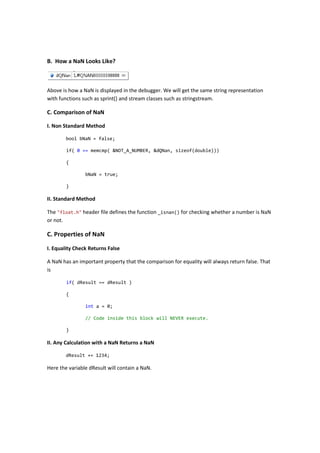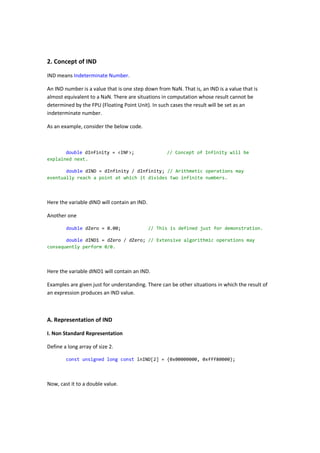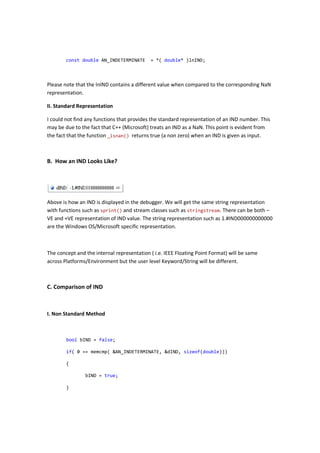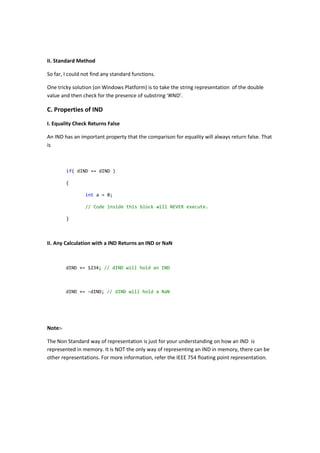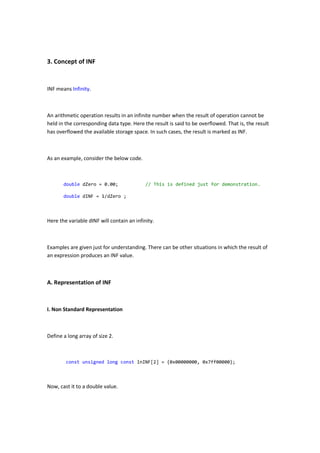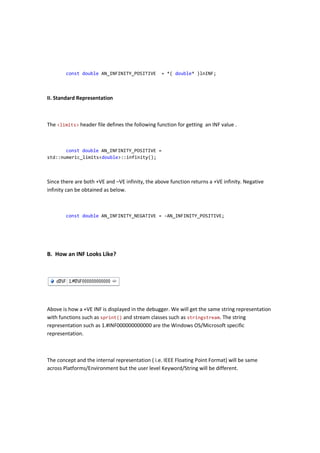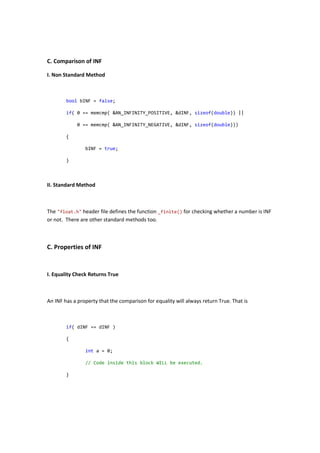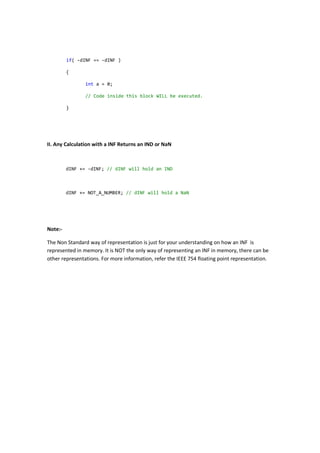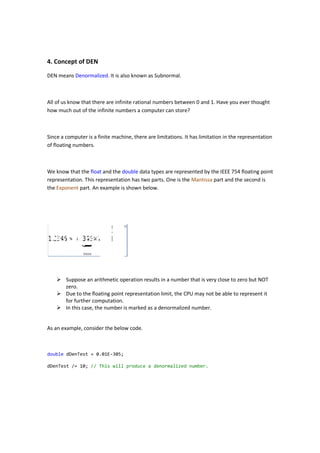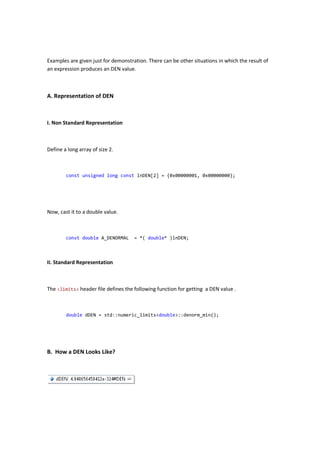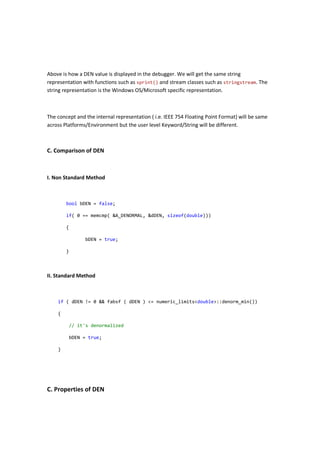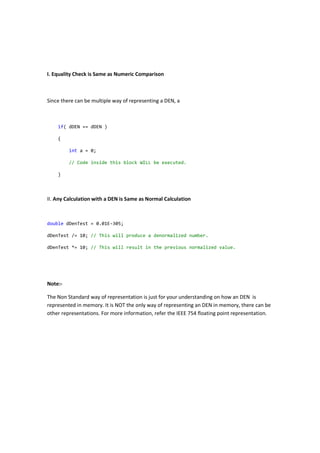Próximos SlideShares02 Primitive data types and variables
Carregando em ... 3
1 de 1602 Primitive data types and variablesmaznabiliCsharp4 operators and_castsAbed BukhariLesson 17. Pattern 9. Mixed arithmeticPVS-StudioC Programming AssignmentVijayananda MohireAssignment c programmingIcaii InfotechJava中的Float&Double以及Ieee754研究V1.0Zianed Hou

### Concept_of_NAN_IND_INF_DEN_Using_C++

1. Numerical Concepts of NaN, IND, INF and DEN Mohammed Nisamudheen S Project Lead UVJ Technologies
2. I would like to share some concepts (some of you might know it already) that C++ offers when dealing floating point arithmetic. 1. Concept of NaN NaN means Not a Number. For an average developer, when something is said to be not a number then it would be a string. This is not the case here. When we perform extensive numerical calculations, the result will be such that it cannot be treated as a number! As an example, consider the below code. double dSQRTValue = sqrt( -1.00 ); // An image processing algorithm may invoke the sqrt() with -1 as its input . double dResult = -dSQRTValue; // A image processing algorithm may involve taking the negative of another value. Here the variable dResult will contain a NaN. So a NaN represents a numeric quantity that cannot be treated as a valid quantity. What can be done to represent it ? Usually we designate 0 or -1 to mark an invalid entry in a float or double variable/array. This kind of idea will not work here because -1 and 0 are valid numbers. A. Representation of NaN I. Non Standard Representation Define a long array of size 2. const unsigned long const lnNAN = {0x00000000, 0x7ff80000}; Now, cast it to a double value! const double NOT_A_NUMBER = *( double* )lnNAN; Now, the constant variable NOT_A_NUMBER contains a NaN. II. Standard Representation The <limits> header file defines the following functions for getting a NaN. const double NOT_A_NUMBER = std::numeric_limits<double>::quiet_NaN();
3. B. How a NaN Looks Like? Above is how a NaN is displayed in the debugger. We will get the same string representation with functions such as sprint() and stream classes such as stringstream. C. Comparison of NaN I. Non Standard Method bool bNaN = false; if( 0 == memcmp( &NOT_A_NUMBER, &dQNan, sizeof(double))) { bNaN = true; } II. Standard Method The "float.h" header file defines the function _isnan() for checking whether a number is NaN or not. C. Properties of NaN I. Equality Check Returns False A NaN has an important property that the comparison for equality will always return false. That is if( dResult == dResult ) { int a = 0; // Code inside this block will NEVER execute. } II. Any Calculation with a NaN Returns a NaN dResult += 1234; Here the variable dResult will contain a NaN.
4. Note:- The Non Standard way of representation is just for your understanding on how a NaN is represented in memory. Please note that it is NOT the only way of representing a NaN in memory, there can be other representations. For more information, we need to refer the IEEE floating point representation.
5. 2. Concept of IND IND means Indeterminate Number. An IND number is a value that is one step down from NaN. That is, an IND is a value that is almost equivalent to a NaN. There are situations in computation whose result cannot be determined by the FPU (Floating Point Unit). In such cases the result will be set as an indeterminate number. As an example, consider the below code. double dInfinity = <INF>; // Concept of Infinity will be explained next. double dIND = dInfinity / dInfinity; // Arithmetic operations may eventually reach a point at which it divides two infinite numbers. Here the variable dIND will contain an IND. Another one double dZero = 0.00; // This is defined just for demonstration. double dIND1 = dZero / dZero; // Extensive algorithmic operations may consequently perform 0/0. Here the variable dIND1 will contain an IND. Examples are given just for understanding. There can be other situations in which the result of an expression produces an IND value. A. Representation of IND I. Non Standard Representation Define a long array of size 2. const unsigned long const lnIND = {0x00000000, 0xfff80000}; Now, cast it to a double value.
6. const double AN_INDETERMINATE = *( double* )lnIND; Please note that the lnIND contains a different value when compared to the corresponding NaN representation. II. Standard Representation I could not find any functions that provides the standard representation of an IND number. This may be due to the fact that C++ (Microsoft) treats an IND as a NaN. This point is evident from the fact that the function _isnan() returns true (a non zero) when an IND is given as input. B. How an IND Looks Like? Above is how an IND is displayed in the debugger. We will get the same string representation with functions such as sprint() and stream classes such as stringstream. There can be both – VE and +VE representation of IND value. The string representation such as 1.#IND000000000000 are the Windows OS/Microsoft specific representation. The concept and the internal representation ( i.e. IEEE Floating Point Format) will be same across Platforms/Environment but the user level Keyword/String will be different. C. Comparison of IND I. Non Standard Method bool bIND = false; if( 0 == memcmp( &AN_INDETERMINATE, &dIND, sizeof(double))) { bIND = true; }
7. II. Standard Method So far, I could not find any standard functions. One tricky solution (on Windows Platform) is to take the string representation of the double value and then check for the presence of substring ‘#IND’. C. Properties of IND I. Equality Check Returns False An IND has an important property that the comparison for equality will always return false. That is if( dIND == dIND ) { int a = 0; // Code inside this block will NEVER execute. } II. Any Calculation with a IND Returns an IND or NaN dIND += 1234; // dIND will hold an IND dIND += -dIND; // dIND will hold a NaN Note:- The Non Standard way of representation is just for your understanding on how an IND is represented in memory. It is NOT the only way of representing an IND in memory, there can be other representations. For more information, refer the IEEE 754 floating point representation.
8. 3. Concept of INF INF means Infinity. An arithmetic operation results in an infinite number when the result of operation cannot be held in the corresponding data type. Here the result is said to be overflowed. That is, the result has overflowed the available storage space. In such cases, the result is marked as INF. As an example, consider the below code. double dZero = 0.00; // This is defined just for demonstration. double dINF = 1/dZero ; Here the variable dINF will contain an infinity. Examples are given just for understanding. There can be other situations in which the result of an expression produces an INF value. A. Representation of INF I. Non Standard Representation Define a long array of size 2. const unsigned long const lnINF = {0x00000000, 0x7ff00000}; Now, cast it to a double value.
9. const double AN_INFINITY_POSITIVE = *( double* )lnINF; II. Standard Representation The <limits> header file defines the following function for getting an INF value . const double AN_INFINITY_POSITIVE = std::numeric_limits<double>::infinity(); Since there are both +VE and –VE infinity, the above function returns a +VE infinity. Negative infinity can be obtained as below. const double AN_INFINITY_NEGATIVE = -AN_INFINITY_POSITIVE; B. How an INF Looks Like? Above is how a +VE INF is displayed in the debugger. We will get the same string representation with functions such as sprint() and stream classes such as stringstream. The string representation such as 1.#INF000000000000 are the Windows OS/Microsoft specific representation. The concept and the internal representation ( i.e. IEEE Floating Point Format) will be same across Platforms/Environment but the user level Keyword/String will be different.
10. C. Comparison of INF I. Non Standard Method bool bINF = false; if( 0 == memcmp( &AN_INFINITY_POSITIVE, &dINF, sizeof(double)) || 0 == memcmp( &AN_INFINITY_NEGATIVE, &dINF, sizeof(double))) { bINF = true; } II. Standard Method The "float.h" header file defines the function _finite() for checking whether a number is INF or not. There are other standard methods too. C. Properties of INF I. Equality Check Returns True An INF has a property that the comparison for equality will always return True. That is if( dINF == dINF ) { int a = 0; // Code inside this block WILL be executed. }
11. if( -dINF == -dINF ) { int a = 0; // Code inside this block WILL be executed. } II. Any Calculation with a INF Returns an IND or NaN dINF += -dINF; // dINF will hold an IND dINF += NOT_A_NUMBER; // dINF will hold a NaN Note:- The Non Standard way of representation is just for your understanding on how an INF is represented in memory. It is NOT the only way of representing an INF in memory, there can be other representations. For more information, refer the IEEE 754 floating point representation.
12. 4. Concept of DEN DEN means Denormalized. It is also known as Subnormal. All of us know that there are infinite rational numbers between 0 and 1. Have you ever thought how much out of the infinite numbers a computer can store? Since a computer is a finite machine, there are limitations. It has limitation in the representation of floating numbers. We know that the float and the representation. This representation has two parts. One is the the Exponent part. An example is shown below.  Suppose an arithmetic operation results in a number that is very close to zero but NOT zero.  Due to the floating point representation limit, the CPU may not be able to represent it for further computation.  In this case, the number is marked as a denormalized number. As an example, consider the below code. double dDenTest = 0.01E- dDenTest /= 10; // This will produce a denormalized number. . It is also known as Subnormal. All of us know that there are infinite rational numbers between 0 and 1. Have you ever thought out of the infinite numbers a computer can store? Since a computer is a finite machine, there are limitations. It has limitation in the representation and the double data types are represented by the IEEE 754 representation. This representation has two parts. One is the Mantissa part and the second is part. An example is shown below. Suppose an arithmetic operation results in a number that is very close to zero but NOT Due to the floating point representation limit, the CPU may not be able to represent it for further computation. In this case, the number is marked as a denormalized number. As an example, consider the below code. -305; // This will produce a denormalized number. All of us know that there are infinite rational numbers between 0 and 1. Have you ever thought Since a computer is a finite machine, there are limitations. It has limitation in the representation data types are represented by the IEEE 754 floating point part and the second is Suppose an arithmetic operation results in a number that is very close to zero but NOT Due to the floating point representation limit, the CPU may not be able to represent it
13. Examples are given just for demonstration. There can be other situations in which the result of an expression produces an DEN value. A. Representation of DEN I. Non Standard Representation Define a long array of size 2. const unsigned long const lnDEN = {0x00000001, 0x00000000}; Now, cast it to a double value. const double A_DENORMAL = *( double* )lnDEN; II. Standard Representation The <limits> header file defines the following function for getting a DEN value . double dDEN = std::numeric_limits<double>::denorm_min(); B. How a DEN Looks Like?
14. Above is how a DEN value is displayed in the debugger. We will get the same string representation with functions such as sprint() and stream classes such as stringstream. The string representation is the Windows OS/Microsoft specific representation. The concept and the internal representation ( i.e. IEEE 754 Floating Point Format) will be same across Platforms/Environment but the user level Keyword/String will be different. C. Comparison of DEN I. Non Standard Method bool bDEN = false; if( 0 == memcmp( &A_DENORMAL, &dDEN, sizeof(double))) { bDEN = true; } II. Standard Method if ( dDEN != 0 && fabsf ( dDEN ) <= numeric_limits<double>::denorm_min()) { // it's denormalized bDEN = true; } C. Properties of DEN
15. I. Equality Check is Same as Numeric Comparison Since there can be multiple way of representing a DEN, a if( dDEN == dDEN ) { int a = 0; // Code inside this block WILL be executed. } II. Any Calculation with a DEN is Same as Normal Calculation double dDenTest = 0.01E-305; dDenTest /= 10; // This will produce a denormalized number. dDenTest *= 10; // This will result in the previous normalized value. Note:- The Non Standard way of representation is just for your understanding on how an DEN is represented in memory. It is NOT the only way of representing an DEN in memory, there can be other representations. For more information, refer the IEEE 754 floating point representation.
16. This is the last page of this document.